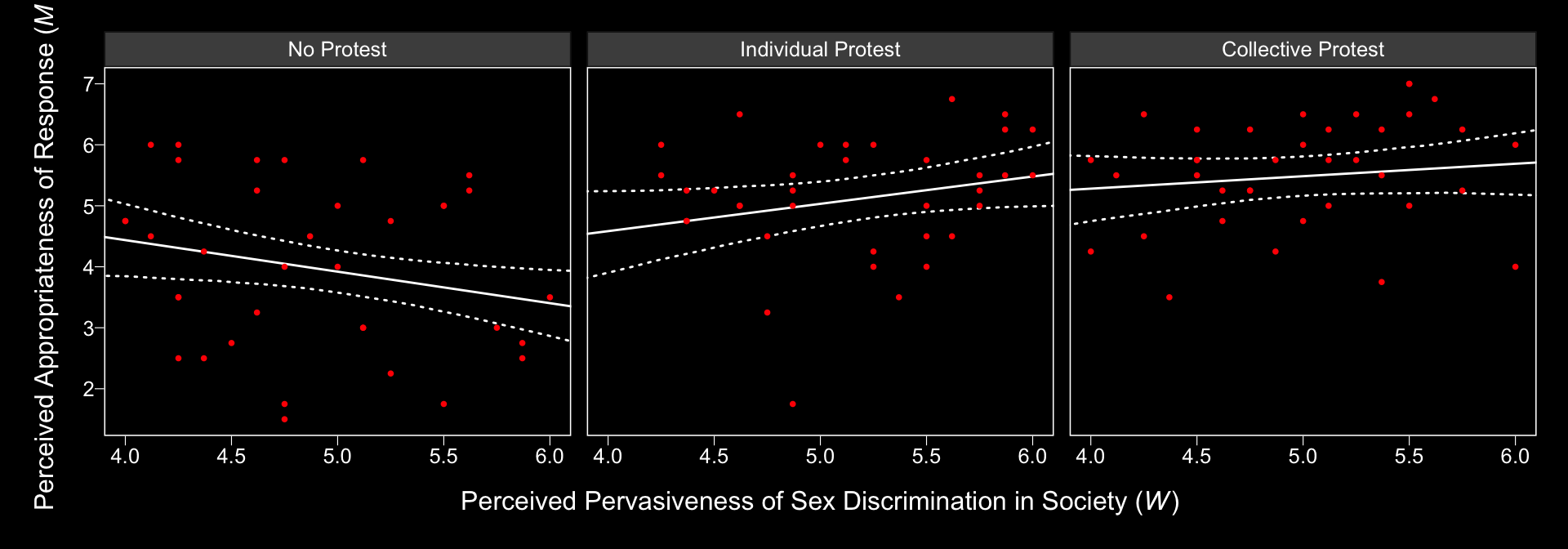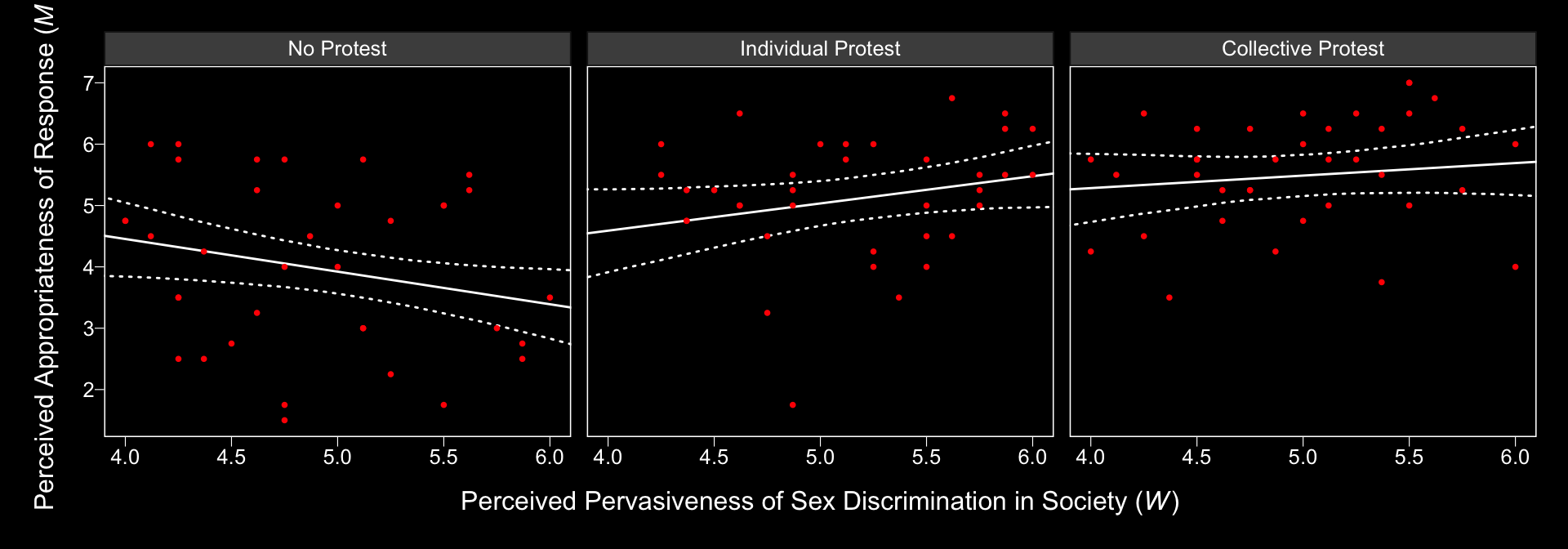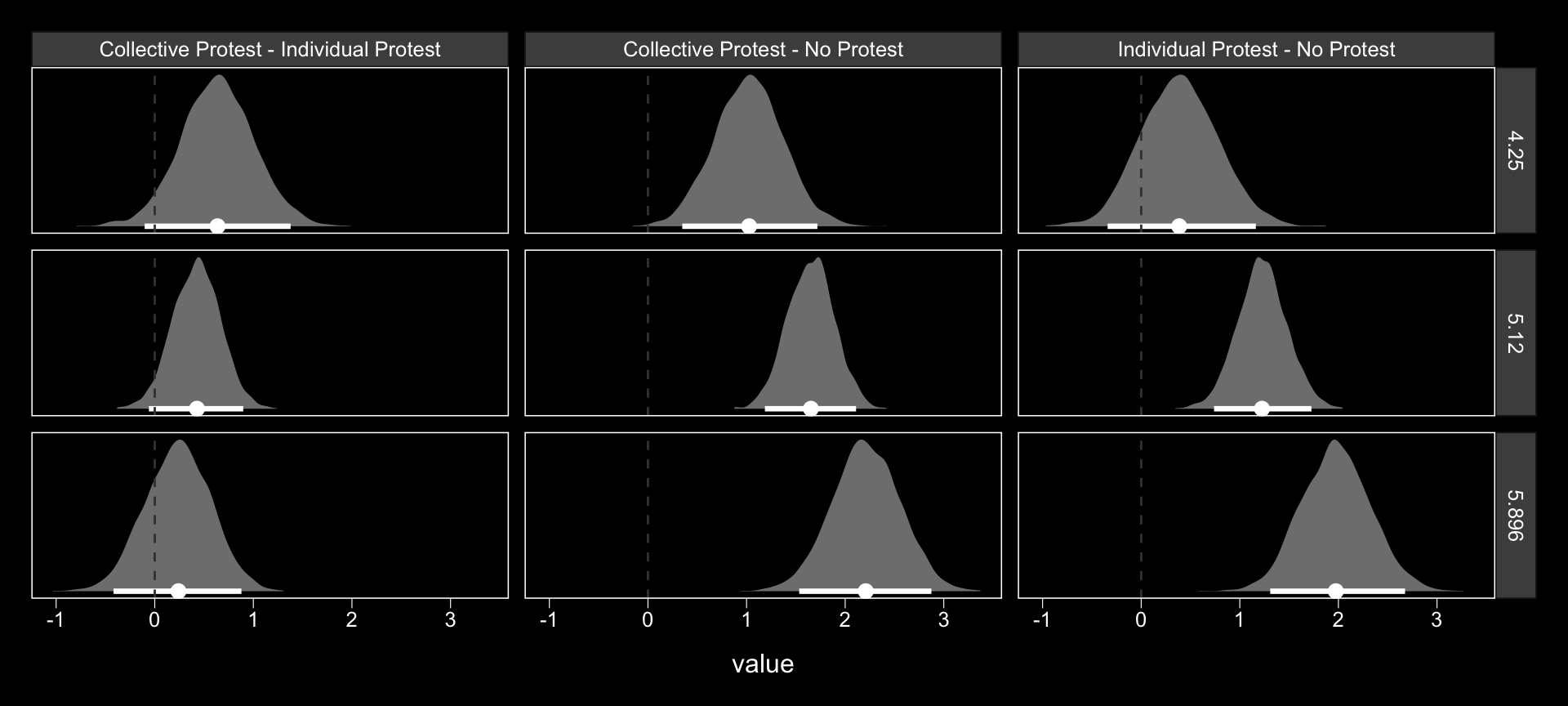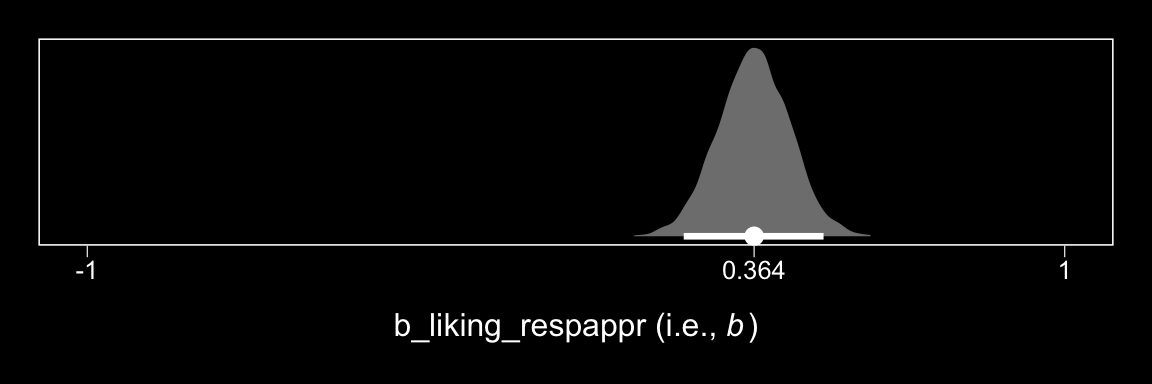## 13.2 Looking at the components of the indirect effect of $$X$$

### 13.2.1 Examining the first stage of the mediation process.

When making a newdata object to feed into fitted() with more complicated models, it can be useful to review the model formula like so:

model1$formula ## respappr ~ 1 + D1 + D2 + sexism + D1:sexism + D2:sexism ## liking ~ 1 + D1 + D2 + respappr + sexism + D1:sexism + D2:sexism Now we’ll prep for and make our version of Figure 13.3. nd <- tibble(D1 = rep(c(1/3, -2/3, 1/3), each = 30), D2 = rep(c(1/2, 0, -1/2), each = 30), sexism = rep(seq(from = 3.5, to = 6.5, length.out = 30), times = 3)) model1_fitted <- fitted(model1, newdata = nd, resp = "respappr") %>% as_tibble() %>% bind_cols(nd) %>% mutate(condition = ifelse(D2 == 0, "No Protest", ifelse(D2 == -1/2, "Individual Protest", "Collective Protest"))) %>% mutate(condition = factor(condition, levels = c("No Protest", "Individual Protest", "Collective Protest"))) protest <- protest %>% mutate(condition = ifelse(protest == 0, "No Protest", ifelse(protest == 1, "Individual Protest", "Collective Protest"))) %>% mutate(condition = factor(condition, levels = c("No Protest", "Individual Protest", "Collective Protest"))) model1_fitted %>% ggplot(aes(x = sexism, group = condition)) + geom_ribbon(aes(ymin = Q2.5, ymax = Q97.5), linetype = 3, color = "white", fill = "transparent") + geom_line(aes(y = Estimate), color = "white") + geom_point(data = protest, aes(x = sexism, y = respappr), color = "red", size = 2/3) + coord_cartesian(xlim = 4:6) + labs(x = expression(paste("Perceived Pervasiveness of Sex Discrimination in Society (", italic(W), ")")), y = expression(paste("Perceived Appropriateness of Response (", italic(M), ")"))) + theme_black() + theme(panel.grid = element_blank()) + facet_wrap(~condition)In order to get the $$\Delta R^2$$ distribution analogous to the change in $$R^2$$ $$F$$-test Hayes discussed on page 482, we’ll have to first refit the model without the interaction for the $$M$$ criterion. Here are the sub-models. m_model <- bf(respappr ~ 1 + D1 + D2 + sexism) y_model <- bf(liking ~ 1 + D1 + D2 + respappr + sexism + D1:sexism + D2:sexism) Now we fit model2. model2 <- brm(data = protest, family = gaussian, m_model + y_model + set_rescor(FALSE), chains = 4, cores = 4) With model2 in hand, we’re ready to compare $$R^2$$ distributions. R2s <- bayes_R2(model1, resp = "respappr", summary = F) %>% as_tibble() %>% rename(model1 = R2_respappr) %>% bind_cols( bayes_R2(model2, resp = "respappr", summary = F) %>% as_tibble() %>% rename(model2 = R2_respappr) ) %>% mutate(difference = model1 - model2) R2s %>% ggplot(aes(x = difference)) + geom_halfeyeh(aes(y = 0), fill = "grey50", color = "white", point_interval = median_qi, .prob = 0.95) + scale_x_continuous(breaks = median_qi(R2s$difference, .prob = .95)[1, 1:3],
labels = median_qi(R2s$difference, .prob = .95)[1, 1:3] %>% round(2)) + scale_y_continuous(NULL, breaks = NULL) + xlab(expression(paste(Delta, italic(R)^2))) + theme_black() + theme(panel.grid = element_blank())And we might also compare the models by their information criteria. loo(model1, model2) ## LOOIC SE ## model1 760.10 29.56 ## model2 765.47 29.71 ## model1 - model2 -5.37 7.96 waic(model1, model2) ## WAIC SE ## model1 759.74 29.47 ## model2 765.32 29.71 ## model1 - model2 -5.58 7.96 The Bayesian $$R^2$$, the LOO-CV, and the WAIC all suggest there’s little difference between the two models with respect to their predictive utility. In such a case, I’d lean on theory to choose between them. If inclined, one could also do Bayesian model averaging. Within our Bayesian modeling paradigm, we don’t have a direct analogue to the $$F$$-tests Hayes presented on page 483. But a little fitted() and follow-up wrangling will give us some difference scores. # we need new nd data nd <- tibble(D1 = rep(c(1/3, -2/3, 1/3), each = 3), D2 = rep(c(1/2, 0, -1/2), each = 3), sexism = rep(c(4.250, 5.120, 5.896), times = 3)) # this time we'll use summary = F model1_fitted <- fitted(model1, newdata = nd, resp = "respappr", summary = F) %>% as_tibble() %>% gather() %>% mutate(condition = rep(c("Collective Protest", "No Protest", "Individual Protest"), each = 3*4000), sexism = rep(c(4.250, 5.120, 5.896), times = 3) %>% rep(., each = 4000), iter = rep(1:4000, times = 9)) %>% select(-key) %>% spread(key = condition, value = value) %>% mutate(Individual Protest - No Protest = Individual Protest - No Protest, Collective Protest - No Protest = Collective Protest - No Protest, Collective Protest - Individual Protest = Collective Protest - Individual Protest) # a tiny bit more wrangling and we're ready to plot the difference distributions model1_fitted %>% select(sexism, contains("-")) %>% gather(key, value, -sexism) %>% ggplot(aes(x = value)) + geom_halfeyeh(aes(y = 0), fill = "grey50", color = "white", point_interval = median_qi, .prob = 0.95) + geom_vline(xintercept = 0, color = "grey25", linetype = 2) + scale_y_continuous(NULL, breaks = NULL) + facet_grid(sexism~key) + theme_black() + theme(panel.grid = element_blank())Now we have model1_fitted, it’s easy to get the typical numeric summaries for the differences. model1_fitted %>% select(sexism, contains("-")) %>% gather(key, value, -sexism) %>% group_by(key, sexism) %>% summarize(mean = mean(value), ll = quantile(value, probs = .025), ul = quantile(value, probs = .975)) %>% mutate_if(is.double, round, digits = 3) ## # A tibble: 9 x 5 ## # Groups: key  ## key sexism mean ll ul ## <chr> <dbl> <dbl> <dbl> <dbl> ## 1 Collective Protest - Individual Protest 4.25 0.636 -0.102 1.38 ## 2 Collective Protest - Individual Protest 5.12 0.424 -0.059 0.898 ## 3 Collective Protest - Individual Protest 5.90 0.234 -0.418 0.879 ## 4 Collective Protest - No Protest 4.25 1.02 0.348 1.72 ## 5 Collective Protest - No Protest 5.12 1.65 1.19 2.11 ## 6 Collective Protest - No Protest 5.90 2.21 1.53 2.87 ## 7 Individual Protest - No Protest 4.25 0.388 -0.34 1.16 ## 8 Individual Protest - No Protest 5.12 1.23 0.739 1.73 ## 9 Individual Protest - No Protest 5.90 1.98 1.31 2.68 The three levels of Collective Protest - Individual Protest correspond nicely with some of the analyses Hayes presented on pages 484–486. However, they don’t get at the differences Hayes expressed as $$\theta_{D_{1}\rightarrow M}$$ to . For those, we’ll have to work directly with the posterior_samples(). post <- posterior_samples(model1) post %>% mutate(Difference in how Catherine's behavior is perceived between being told she protested or not when W is 4.250 = b_respappr_D1 + b_respappr_D1:sexism*4.250, Difference in how Catherine's behavior is perceived between being told she protested or not when W is 5.210 = b_respappr_D1 + b_respappr_D1:sexism*5.120, Difference in how Catherine's behavior is perceived between being told she protested or not when W is 5.896 = b_respappr_D1 + b_respappr_D1:sexism*5.896) %>% select(contains("Difference")) %>% gather() %>% group_by(key) %>% summarize(mean = mean(value), ll = quantile(value, probs = .025), ul = quantile(value, probs = .975)) %>% mutate_if(is.double, round, digits = 3) ## # A tibble: 3 x 4 ## key mean ll ul ## <chr> <dbl> <dbl> <dbl> ## 1 Difference in how Catherine's behavior is perceived between being told she pro… 0.706 0.086 1.34 ## 2 Difference in how Catherine's behavior is perceived between being told she pro… 1.44 1.02 1.85 ## 3 Difference in how Catherine's behavior is perceived between being told she pro… 2.09 1.51 2.69 ### 13.2.2 Estimating the second stage of the mediation process. Here’s $$b$$. post %>% ggplot(aes(x = b_liking_respappr)) + geom_halfeyeh(aes(y = 0), fill = "grey50", color = "white", point_interval = median_qi, .prob = 0.95) + scale_x_continuous(breaks = c(-1, median(post$b_liking_respappr), 1),
labels = c(-1,
median(post\$b_liking_respappr) %>% round(3),
1)) +
scale_y_continuous(NULL, breaks = NULL) +
coord_cartesian(xlim = -1:1) +
xlab(expression(paste("b_liking_respappr (i.e., ", italic(b), ")"))) +
theme_black() +
theme(panel.grid = element_blank())